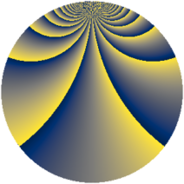# Properties

 Label 552.2.uLevel $552$ Weight $2$ Character orbit 552.u Rep. character $\chi_{552}(17,\cdot)$ Character field $\Q(\zeta_{22})$ Dimension $240$ Newform subspaces $1$ Sturm bound $192$ Trace bound $0$

# Related objects

## Defining parameters

 Level: $$N$$ $$=$$ $$552 = 2^{3} \cdot 3 \cdot 23$$ Weight: $$k$$ $$=$$ $$2$$ Character orbit: $$[\chi]$$ $$=$$ 552.u (of order $$22$$ and degree $$10$$) Character conductor: $$\operatorname{cond}(\chi)$$ $$=$$ $$69$$ Character field: $$\Q(\zeta_{22})$$ Newform subspaces: $$1$$ Sturm bound: $$192$$ Trace bound: $$0$$

## Dimensions

The following table gives the dimensions of various subspaces of $$M_{2}(552, [\chi])$$.

Total New Old
Modular forms 1040 240 800
Cusp forms 880 240 640
Eisenstein series 160 0 160

## Trace form

 $$240q - 4q^{9} + O(q^{10})$$ $$240q - 4q^{9} - 16q^{25} + 12q^{27} - 8q^{31} + 44q^{37} + 20q^{39} + 44q^{43} + 124q^{49} + 12q^{55} + 16q^{69} - 74q^{75} - 144q^{81} + 24q^{85} - 170q^{87} + 12q^{93} - 198q^{99} + O(q^{100})$$

## Decomposition of $$S_{2}^{\mathrm{new}}(552, [\chi])$$ into newform subspaces

Label Dim. $$A$$ Field CM Traces $q$-expansion
$$a_2$$ $$a_3$$ $$a_5$$ $$a_7$$
552.2.u.a $$240$$ $$4.408$$ None $$0$$ $$0$$ $$0$$ $$0$$

## Decomposition of $$S_{2}^{\mathrm{old}}(552, [\chi])$$ into lower level spaces

$$S_{2}^{\mathrm{old}}(552, [\chi]) \cong$$ $$S_{2}^{\mathrm{new}}(69, [\chi])$$$$^{\oplus 4}$$$$\oplus$$$$S_{2}^{\mathrm{new}}(138, [\chi])$$$$^{\oplus 3}$$$$\oplus$$$$S_{2}^{\mathrm{new}}(276, [\chi])$$$$^{\oplus 2}$$